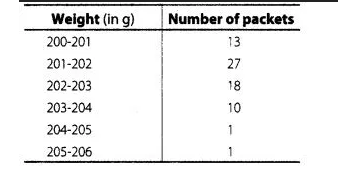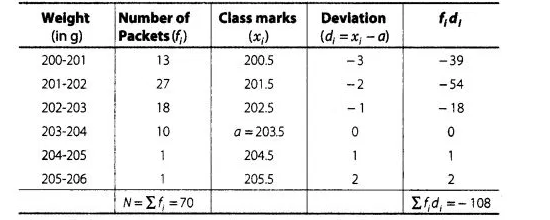# The weights of tea in 70 packets are shown

Question:

The weights of tea in 70 packets are shown in the following tableFind the mean weight of packets.

Solution:

First,we find the class marks of the given data as follows,Here, (assume mean) $a=203.5$

and (class width) $\quad h=1$

By assumed mean method,

$\operatorname{Mean}(\bar{x})=a+\frac{\Sigma f d_{i}}{\sum f_{i}}$

$=203.5-\frac{108}{70}$

$=203.5-1.54=201.96$

Hence, the required mean weight is 201.96 g